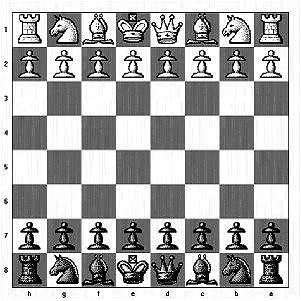시간 제한 메모리 제한 제출 정답 맞은 사람 정답 비율
5 초 512 MB 1 1 1 100.000%

문제

Given an arranged chess board with pieces, figure out the total number of different ways in which any piece can be killed in one move. Note: in this problem, the pieces can be killed despite of the color.For example, if there are 3 pieces King is at B2, Pawn at A1 and Queen at H8 then the total number of pieces that an be killed is 3. H8-Q can kill B2-K, A1-P can kill B2-K, B2-K can kill A1-P

A position on the chess board is represented as A1, A2... A8,B1.. H8

Pieces are represented as

• (K) King can move in 8 direction by one place.
• (Q) Queen can move in 8 direction by any number of places, but can't overtake another piece.
• (R) Rook can only move vertically or horitonzally, but can't overtake another piece.
• (B) Bishop can only move diagonally, but can't overtake another piece.
• (N) Knights can move to a square that is two squares horizontally and one square vertically OR one squares horizontally and two square vertically.
• (P) Pawn can only kill by moving diagonally upwards (towards higher number i.e. A -> B, B->C and so on).

입력

The first line of the input gives the number of test cases, T. T Test cases follow. Each test case consists of the number of pieces , N. N lines follow, each line mentions where a piece is present followed by - with the piece type

Limits

• 1 ≤ T ≤ 100.
• 1 ≤ N ≤ 64.

출력

For each test case, output one line containing "Case #x: y", where x is the test case number (starting from 1) and y is the the total number of different ways in which any piece can be killed.

2
2
A1-K
A8-Q

3
B2-K
A1-P
H8-Q

Case #1: 1
Case #2: 3

채점

• 예제는 채점하지 않는다.